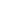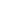info@kalabiz.com7769040506

# Electrical Power CalculatorsThe following calculators are provided to help you determine the size of generator required for your specific application. Other calculators on this page are for unit conversions and other power related calculations.

 Guide to Standard Uints Kilo Volt Amperes kVA KiloWatts (1000 watts = 1 kW) kW Ampere (Volt-Amperes or Current) I Volts E Power Factor PE Percent Efficiency %EFF Horse Power HP

Power Requirement Calculator
 Phase Volts Required V Amperes I Power Factor = Power kW 1 3 .8 1.0

Converting kW to kVA
 kW = kVA
Converting kVA to kW
 kVA = kW

Converting kW to HP
 kW = HP
What size genset is needed to start a 3 phase electric
motor Direct on Line (DOL) start
 HP of Motor = Generator kVA Required

Calculating Amperes (when you know kVA)
 Phase 1,2,3 Generator kVA Volts Required = Ampere I

3 Phase (415 volt @ 50Hz)
1 Phase (240 volt @ 50Hz)

Starting Sequence Motor HP Start Method DOL / StarDelta Run Variable Phase Current Starting Value Total Run Current Total Start Current
1 /
2 /
3 /
4 /
5 /
6 /
 Generator Size for Starting with: kVA* Generator Size for Running with: kVA* Generator Size Recommended: kVA*

NOTE : Above calculations are an estimate only because other factors such
as site conditions, motor efficiencies and iron losses are not taken paper writers into consideration.